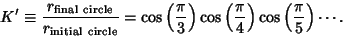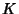## Polygon Inscribing Constant

If a Triangle is inscribed in a Circle, another Circle inside the Triangle, a Square inside the Circle, another Circle inside the Square, and so on,Numerically,whereis the Polygon Circumscribing Constant. Kasner and Newman's (1989) assertion thatis incorrect.

Let a convex Polygon be inscribed in a Circle and divided into Triangles from diagonals from one Vertex. The sum of the Radii of the Circles inscribed in these Triangles is the same independent of the Vertex chosen (Johnson 1929, p. 193).

References

Finch, S. Favorite Mathematical Constants.'' http://www.mathsoft.com/asolve/constant/infprd/infprd.html

Johnson, R. A. Modern Geometry: An Elementary Treatise on the Geometry of the Triangle and the Circle. Boston, MA: Houghton Mifflin, 1929.

Kasner, E. and Newman, J. R. Mathematics and the Imagination. Redmond, WA: Microsoft Press, pp. 311-312, 1989.

Pappas, T. Infinity & Limits.'' The Joy of Mathematics. San Carlos, CA: Wide World Publ./Tetra, p. 180, 1989.

Plouffe, S. Product(cos(Pi/n),n=3..infinity).'' http://www.lacim.uqam.ca/piDATA/productcos.txt.